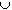#Interactive Real Analysis

Next | Previous | Glossary | Map

## 1.1. Notation and Set Theory

### Examples 1.1.2(c):

• E = { x: x = 2n for nN }
• O = {x : x = 2n - 1 for nN }
• I = {xR: x * x = -2}
Find the sets OE, OI, comp(I).
• OE is the set of all natural numbers that are even or odd. Hence OE = N.

• OE is the set of all natural numbers that are both even and odd. Hence OE = 0 (empty set)

• comp(I) is the set of all numbers that are not in I. Since I is empty, it is the set of numbers that are not in the empty set. Hence: comp(I) = R (here again the universe is understood to be all real numbers).
Next | Previous | Glossary | Map